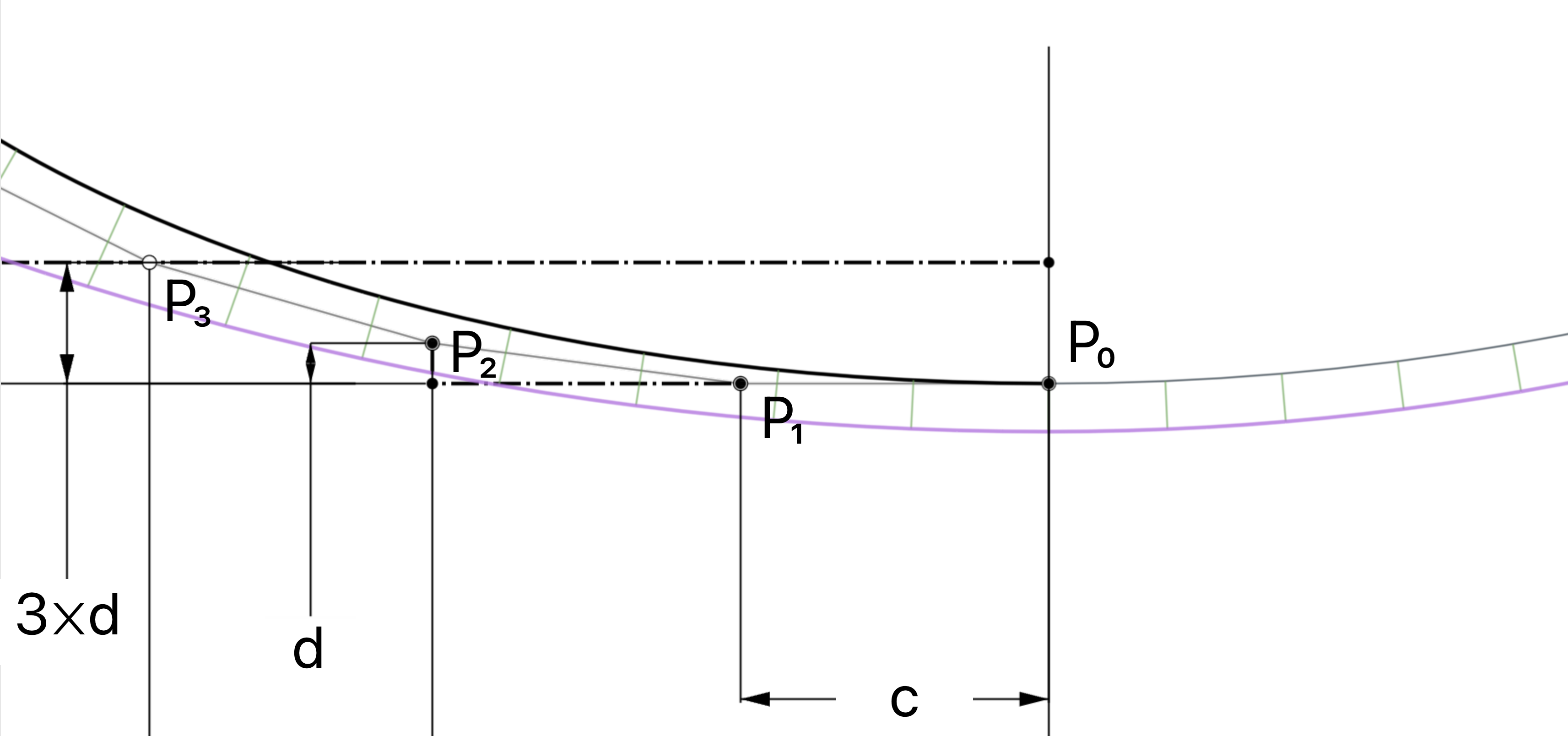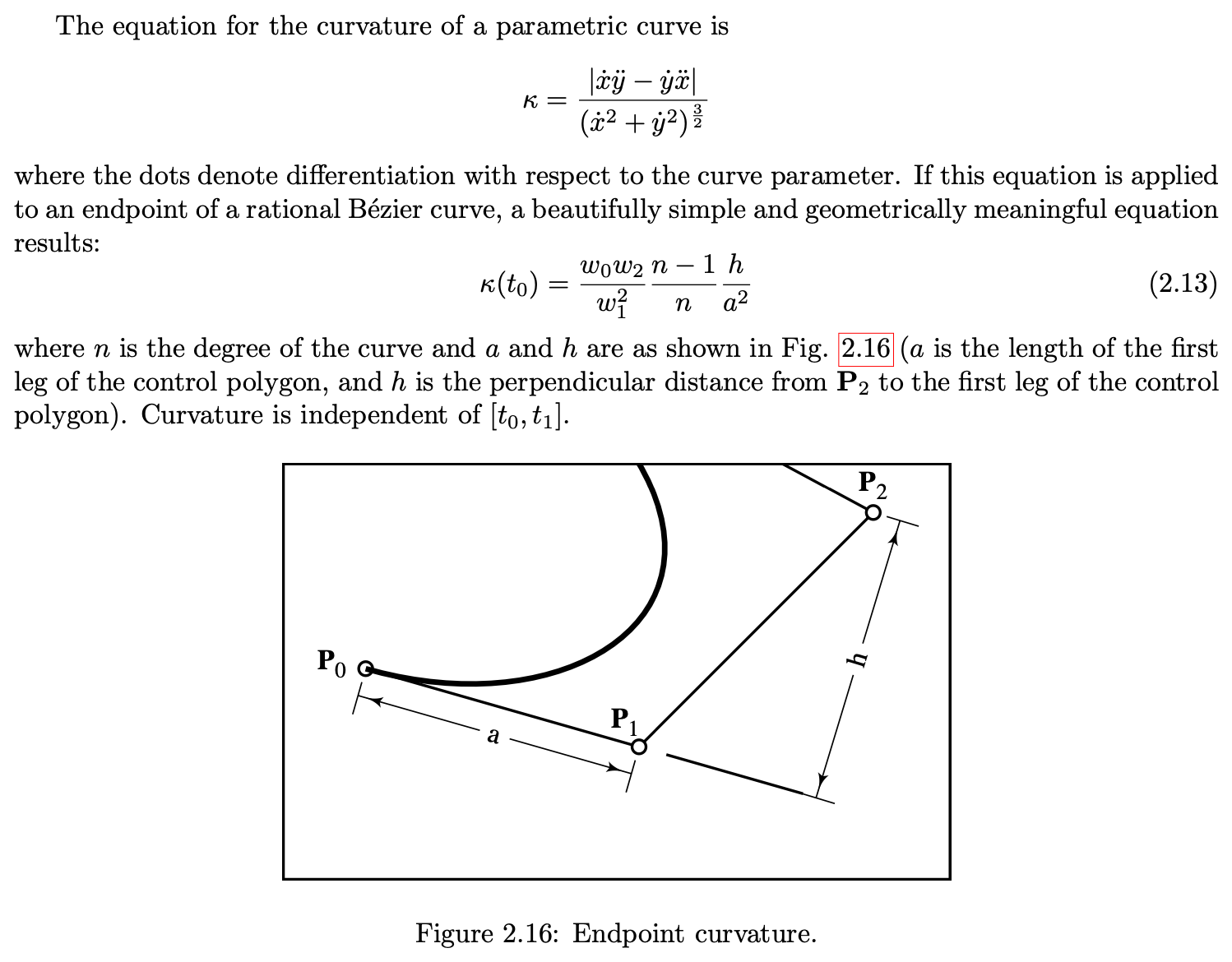Welcome to the Onshape forum! Ask questions and join in the discussions about everything Onshape.

First time visiting? Here are some places to start:
1. Looking for a certain topic? Check out the categories filter or use Search (upper right).
2. Need support? Ask a question to our Community Support category.
3. Please submit support tickets for bugs but you can request improvements in the Product Feedback category.
4. Be respectful, on topic and if you see a problem, Flag it.

If you would like to contact our Community Manager personally, feel free to send a private message or an email.

# G³ Constraint for Bézier?

Given a Bézier which is degree n (and in this case n≥3), if I want to make the P₀ end point G² to another curve, I know that there's a simple equation. Let c= distance from P₀ to P₁, d= normal distance of P₂ from the P₀-P₁ vector, and 𝜅 is the curvature of the adjoining curve at the common endpoint. Then d=(n/(n-1))𝜅c². Is there a similar simple equation for a G³ constraint for the P₃ point?

From what I've seen so far, if the adjoining curve has constant curvature (i.e. jolt is 0), then P₃ lies on a line 3d from the P₀-P₁ vector.Tagged:

• On further experimentation, it seems P₃ works like this iff P₂ is 2⨉c from P₀. Otherwise the 3⨉d dimension must also change. I don't yet have that relationship worked out.
• Watch this video. the answer is probably in it somewhere at the end. She explains up to G3 and C3 and how to achieve it

You can also look how the bridging curve code extracts G2 continuity.

what possible reason do you have to need G3 control?  That is going to create one complex curve.
• edited December 2022
I'm pretty familiar with Freya's work - I was one of a very large group of Patreons that supported this work. I posted this video here recently. She does a good job explaining parametric vs geometric continuity and some stuff about what it takes to achieve each. However, if I'm trying to make a new curve G³ to two existing curves/edges, those driving curves may not also be Béziers. I can extract the curvature at the end point using evEdgeCurvatures(), but there's no built in tool to extract the jolt. Extracting the control points, knots, and weights of an arbitrary NURBS curve is not really helpful given the mathematical complexity of anything other than a Bézier.

G³ (and sometimes higher) are necessary to do class-A surfacing for industrial design. Rhino, Creo, Alias, Icem, Solidworks, Nx, Catia, etc all have G³ constraints. If you want the zebra stripes to be ≥G², you need the surfaces to be ≥G³.
• Freya is great. I watched that video 2x.

I have never come across any code to even extract the G2 continuity let alone the jolt.  Hopefully someone on here has and can supply it to us.
I think you need to establish some sort of rules for your requirement.

Another option is to import a curve from one of the other systems and import it and analyze how that cad engine decided to generate that curve.  If it does not import as a BSpline curve and needs to be analyzed using evApproximateBSplineCurve, then export it as a step file(just the curve) and you can read the b spline curve data in the step file by opening it with notepad.  Then reverse engineer it somehow to determine how they determine where the control points are placed.

If you don't have access to any of those cad systems, which i dont either, then hopefully someone can give you a step file with the curves you need to reverse engineer it.

• edited December 2022
G2 is in Bridging curve.

```    // using k = ((degree - 1) / degree) * h / |t| ^ 2, where k is curvature, t is tangent vector, h is the minimum distance
// from the next control point to the extension of tangent direction
var distance = (degree / (degree - 1)) * curvatureFrame.curvature * speed * speed;```

Below is from Thomas W Sederberg's Computer Aided Geometric Design (a free-to-download 273 page book which is a great reference on Béziers, B-Splines, etc). Assuming that we're using non-rational Béziers, you can ignore the first term in the 2.13 equation, and that's the same as what's in the Bridging curve code. "speed" is a in the diagram below, "distance" is h. The Bridging curve equation has just solved 2.13 for h instead of k.